# Neoclassical Viscosity Matrices

The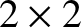dimensionless species-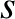neoclassical viscosity matrix,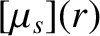(see Section 2.17), is defined to have the following elements :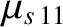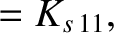(A.53)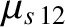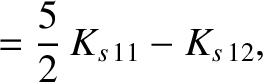(A.54)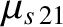(A.55)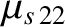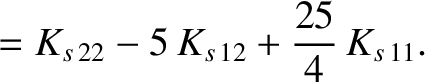(A.56)

(Note that the viscosity matrix elements defined here differ from those defined in Section 2.17 by a factor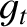.) Here,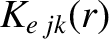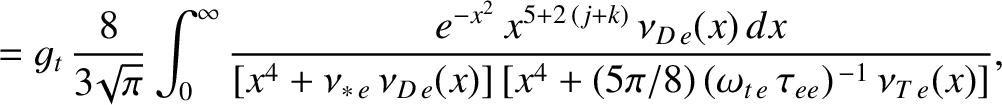(A.57)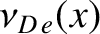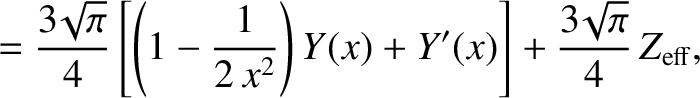(A.58)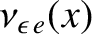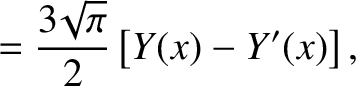(A.59)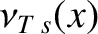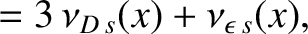(A.60)

and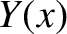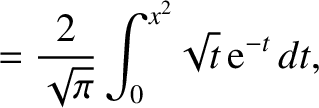(A.61)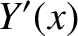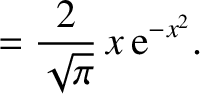(A.62)

Furthermore,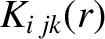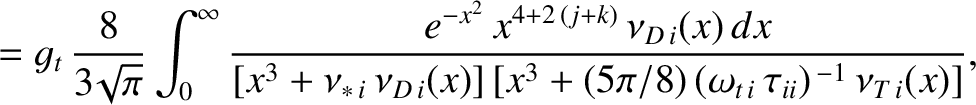(A.63)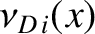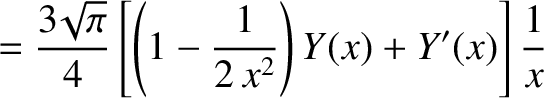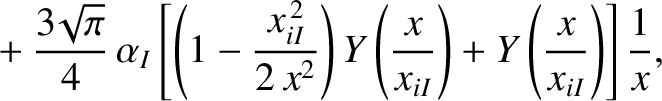(A.64)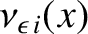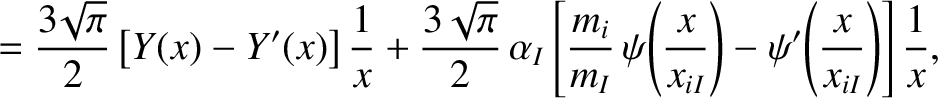(A.65)

and, finally,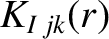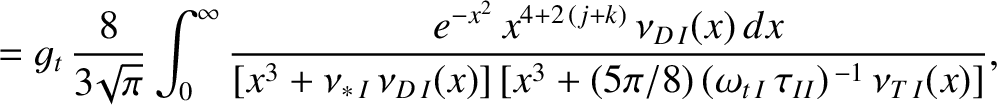(A.66)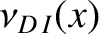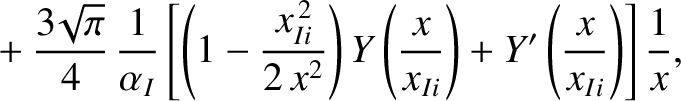(A.67)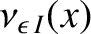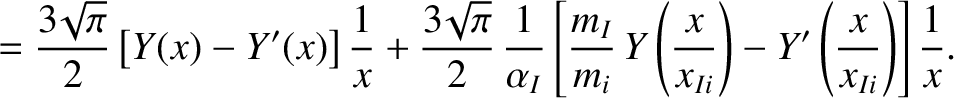(A.68)

Note that our expressions for the neoclassical viscosity matrices interpolate in the most accurate manner possible between the three standard neoclassical collsionality regimes (i.e., the banana, plateau, and Pfirsch-Schlüter regimes ) .